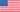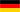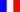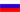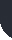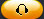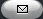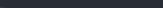Task Management Software for Team Collaboration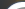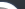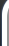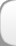HomeDownloadSupportPurchase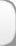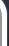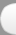Installation GuideStartUp GuideOnline Help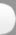# Actual Labor Rate Custom Field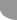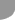Home  » Products  » VIP Task Manager Pro  » Tutorials  » Customization Guide  » Actual Labor Rate Custom Field

Actual Labor Rate

Actual Labor Rate is the actual amount paid to employee per each direct labor hour. Actual Labor Rate can be computed using the formula below:

Actual Labor Rate = Direct Labor Cost / Direct Labor Hours of all direct labor employees

Direct Labor Hours of all direct labor employees = Number of hours per day of each employee * Number of working days per each employee * Number of direct labor  employees

Assume that you have 45 employees and Direct Labor Cost is \$60 000 USD. Firstly, you need to calculate how many hours each employee worked. If you have standard capacity of 40 hours of work per week and you need to calculate Direct Labor Hours of all employees per 2-week period, total number of Direct Labor hours of all 45 direct labor employees is calculated like this:

Direct Labor Hours of all employees = 8 hours * 5 working days * 2 weeks * 45 employees = 3600 hours

Actual Labor Rate is calculated dividing Direct Labor Cost by Direct Labor Hours of all direct labor employees.

Actual Labor Rate = \$60 000 USD / 3600 hours = \$16.67 USD

Actual Labor Rate shows how much a company actually spends to hire employee per direct labor hour.

• Add custom field Total Direct Labor Cost with Code cf_total_direct_labor_cost
•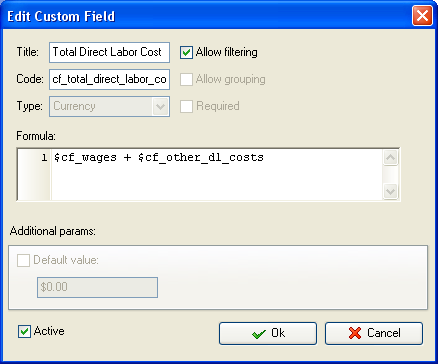Add wages and other costs associated with direct labor (pension contributions, employee insurance, payroll tax and other direct labor costs)

• Add custom field Number of hours per day with Code cf_number_of_hours_per_day
•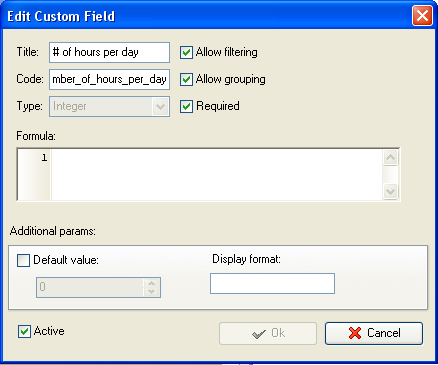• Add custom field Number of working days per week with Code cf_number_of_working_days
•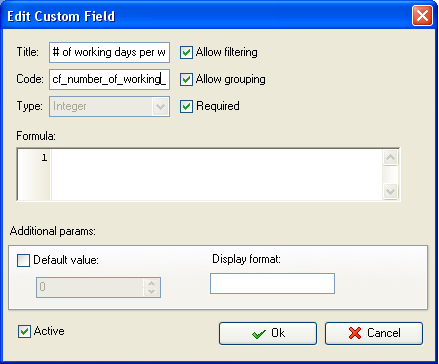• Add custom field Direct Labor Hours  with Code cf_direct_labor_hours and formula:
• \$cf_number_of_hours_per_day * \$cf_number_of_working_days * X * Y

Where X is the number of weeks within which you need to compute Actual Labor Rate. According to the example above, the measurement period is 2 weeks.

Y is the number of employees. In our example we calculated Actual Labor Rate for 45 employees.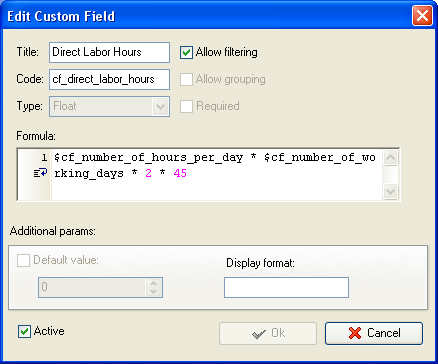Note: you can create one custom field Direct Labor Hours to enter the total number of direct labor hours for all employees manually (e.g. 3600 hours) or add custom field with the following formula to calculate Direct Labor Hours for all employees:

Number of hours per day * Total Number of working days * Number of direct labor employees

Keeping with the example above:

Number of hours per day  8,

Total Number of working days = 5 working days per week * 2-week measurement period = 10 days

Number of direct labor employees  45

The formula for this custom field can look like this:

\$cf_number_of_hours_per_day * \$cf_total_number_of_working_days * X, where

X is the number of employees

• Add custom field Actual Labor Rate with Code cf_actual_labor_rate and formula:
• \$cf_total_direct_labor_cost / \$cf_direct_labor_hours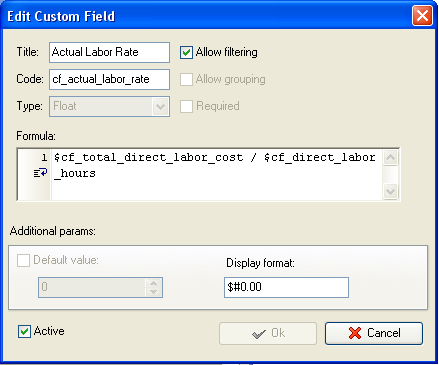• On Task Tree select task group for which you need to display Actual Labor Rate and select Custom fields options
•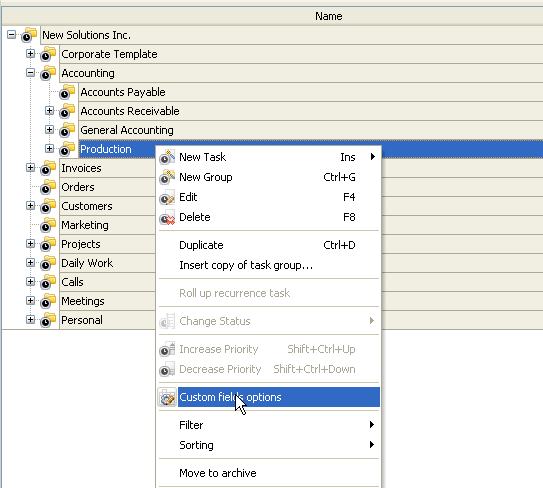• To fill in custom fields Number of Hours per day, Number of working hours per week, Direct Labor Hours (if no formula is used) and/or any custom field used to calculate Direct Labor Hours at task group level, set the following custom fields settings for these custom fields:
• In Settings for select This task group
• select Enable
• select Edit
•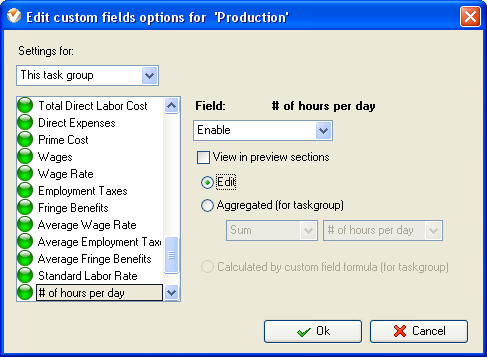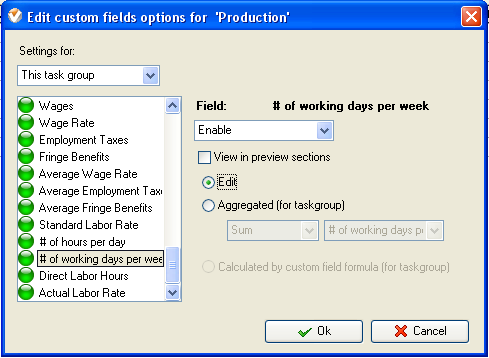• To fill in the custom field at the task group right click on task group, select Custom fields options and select Edit for the corresponding custom field
•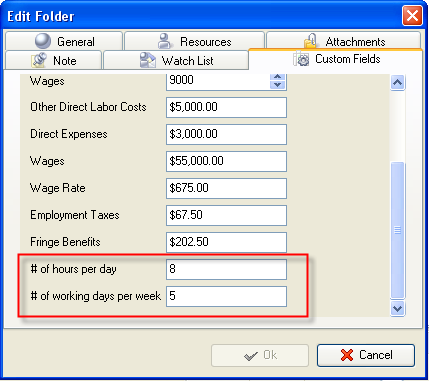• For the custom fields Total Direct Labor Cost, Direct Labor Hours (if the custom field is calculated using the formula) and Actual Labor Rate set the following settings:
• In Settings for select This task group
• select Enable
• select Calculated by custom field formula (for task group)
•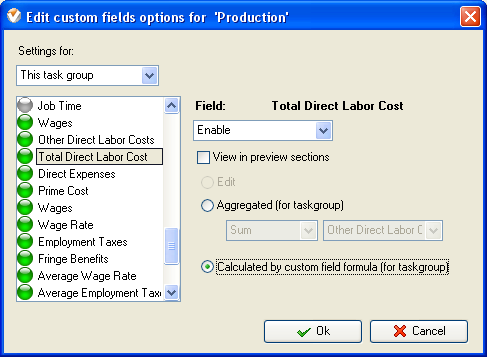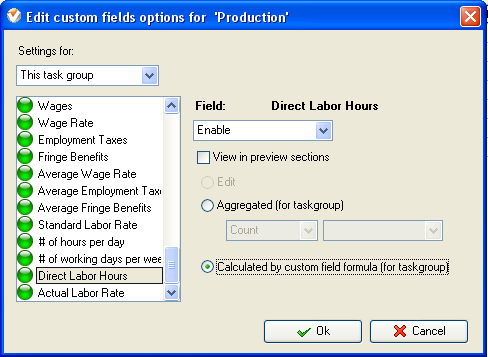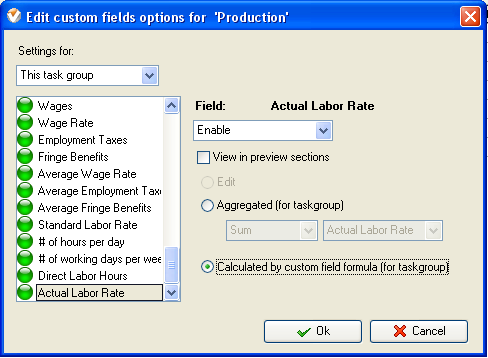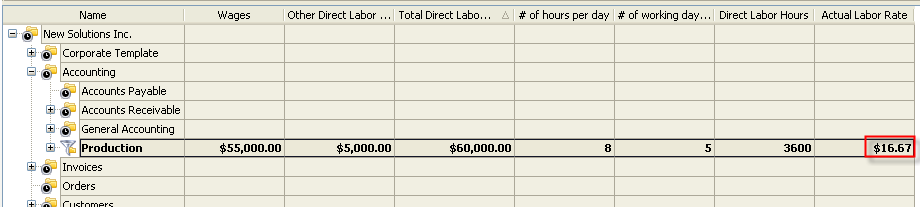Actual Labor Rate on Task Tree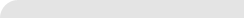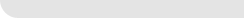» VIP Task Manager Pro VIP Task Manager Std Testimonials Turnkey Solution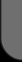ProductsSolutionsDownloadSupportPurchaseContactsForumVideoMy Account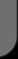Copyright © 2004 - 2023 Task Management Software